﻿ 基于WSN的受限空间逃生路径搜索算法文章快速检索 高级检索
 重庆邮电大学学报(自然科学版)2020, Vol. 32Issue (3): 477-486  DOI: 10.3979/j.issn.1673-825X.2020.03.0180

### 引用本文LI Shuo, GUO Tiancheng. Restricted space escape path search algorithm based on WSN[J]. Journal of Chongqing University of Posts and Telecommunications (Natural Science Edition), 2020, 32(3): 477-486.   DOI: 10.3979/j.issn.1673-825X.2020.03.018.### Foundation item

The National Natural Science Foundation of China(61502055)

.

### 文章历史

Restricted space escape path search algorithm based on WSN
LI Shuo, GUO TianchengSchool of Electrical & Information Engineering, Changsha University of Science and Technology, Changsha 410114, P.R.China
Abstract: Aiming at the rescue or escape of trapped people after a confined environmental accident such as an underground mine or tunnel, this paper proposes a trusted anchor node selection algorithm through constructing a wireless sensor network based on hybrid channel model to realize the accurate location estimation of wireless sensor network nodes in sparse anchor node environment after an accident, generate the optimal escape rescue path, and realize real-time navigation. The simulation results show that the algorithm has higher node positioning accuracy than the existing research in the sparse anchor node scenario, and can accurately search the rescue or escape path based on the dynamic obstacle recognition.
Keywords: wireless sensor network    underground wireless communication    disaster relief    route search    location algorithm
0 引言

Range-free算法则无需距离和角度信息就能实现定位，常见的Range-free定位算法有基于距离矢量计算跳数(distance vector-hop, DV-Hop)算法，凸规划，基于多维标度定位算法(multidimensional scaling, MDS)等。Range-free算法在面对各向同性网络时有着很高的准确性，并且无需额外的硬件支持。文献在DV-Hop算法的基础上预先设置max-hop，当未知节点接收到的锚节点跳数信息大于max-hop，则忽略该锚节点的信息。该算法能够更快更准估计网络，相对DV-Hop算法有着更高的精度。文献提出了一种选择可信锚节点的定位算法，算法主要寻找最小跳跃长度来近似锚节点与节点之间的距离，并且将定位完成的节点升级成锚节点来降低锚节点密度。实验表明，通过使用可信锚节点可以提高定位精度。若将Range-free算法应用到受限空间，受多种因素的影响，往往无法达到理想的精度，并且面对锚节点稀疏的网络时，Range-free算法的定位精度很低。

1) 提出了地下矿井、隧道等受限空间逃生或救援路径搜索的基本框架，为此类场景下实现以安全预警和逃生救援为目标的无线传感器网络部署提供了参考。

2) 建立了无线传感器网络的混合信道模型，通过该模型可实现复杂乃至动态环境下的障碍物检测，构建自动避障的网络拓扑，完成多条可能逃生路径的快速搜索。同时，根据障碍物的检测结果及接收信号强度，该模型可实现节点间距离的精确估计。

3) 设计了可信锚节点选择算法，结合混合信道模式，实现稀疏锚节点环境中无线传感器网络节点的精确位置估计，以确定最优的逃生救援路径。最后，采用文献提出的历史信息定位算法解决逃生导航的移动节点定位问题。表 1 符号以及其意义 Tab.1 Symbol and its meaning
1 基于WSN的逃生救援路径搜索框架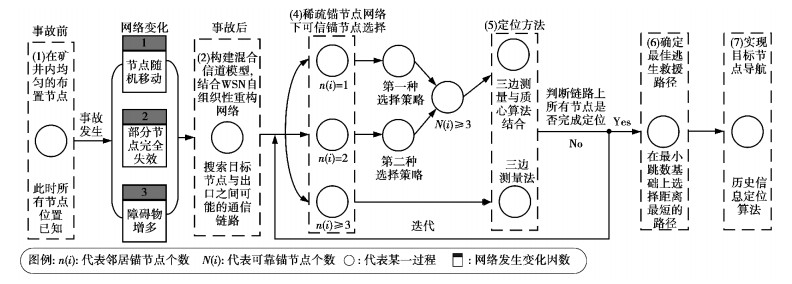图 1 基于混合信道模型无线传感器网络的逃生救援路径搜索框图 Fig.1 Block diagram of escape rescue path search based on hybrid channel model wireless sensor network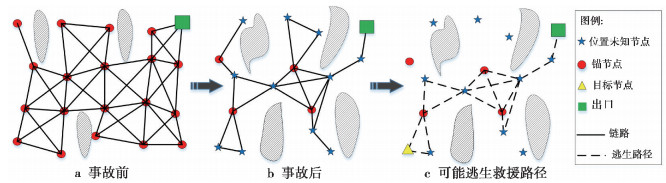图 2 事故前后网络拓扑变化及可能逃生救援路径 Fig.2 Network topology changes before and after the accident and possible escape routes

1) 未发生事故前见图 2a，所有节点位置被认为是已知的。

2) 发生事故后，环境及网络发生以下变化：①部分节点失效(节点密度降低)；②环境中障碍的数量或形状发生变化；③大部分节点的位置改变，少量位置不变的节点为锚节点，形成稀疏锚节点场景，见图 2b

3) 基于混合信道模型的无线传感器网络利用自组织性对网络进行重构，从而确定所有可能的逃生救援路径，见图 2c。通过节点位置的精确估计实现最优的逃生或救援路径选择。

2 混合信道模型

2.1 障碍检测

 $\Delta {\phi _{{\rm{Thr}}}} = \frac{{\sum\limits_{k = 1}^{30} \Delta {\phi _k}}}{{30}}$ (1)

2.2 基于混合信道模型距离估计

 ${L_{\rm{p}}}(d) = {L_{\rm{p}}}({d_0}) + 10{n_{\rm{p}}}\left( {\frac{d}{{{d_0}}}} \right) + {X_\sigma }$ (2)

(2) 式中：d为传播距离(单位：m)；d0为参考距离；np表示发送端与接收端的路径损耗指数；Lp(d0)为距离d0处的信号强度；Xσ服从均值0，标准差为σ2的高斯分布。

 ${L_{\rm{p}}}(d) = {L_{\rm{p}}}({d_0}) + 10{n_{\rm{p}}}\left( {\frac{d}{{{d_0}}}} \right) + {X_\sigma } + \sum\limits_{m = 1}^{{N_{\rm{b}}}} {{\omega _m}}$ (3)

(3) 式中：Nb代表隔墙层数；ωm表示第m个隔墙的衰减因子。通过确定墙壁的衰减因子，可以计算出发射与接收节点之间的距离。

 $\left\{ \begin{array}{l} {L_{\rm{p}}}(d) = {L_{\rm{p}}}({d_0}) + 10{n_{\rm{p}}}\left( {\frac{d}{{{d_0}}}} \right) + {X_\sigma },\Delta \phi \le \Delta {\phi _{{\rm{Thr}}}}\\ {L_{\rm{p}}}(d) = {L_{\rm{p}}}({d_0}) + 10{n_{\rm{p}}}\left( {\frac{d}{{{d_0}}}} \right) + {X_\sigma } + \sum\limits_{m = 1}^{{N_{\rm{b}}}} {{\omega _m}} ,\\ {\kern 1pt} {\kern 1pt} {\kern 1pt} {\kern 1pt} {\kern 1pt} {\kern 1pt} {\kern 1pt} {\kern 1pt} {\kern 1pt} {\kern 1pt} {\kern 1pt} {\kern 1pt} {\kern 1pt} {\kern 1pt} {\kern 1pt} {\kern 1pt} {\kern 1pt} {\kern 1pt} {\kern 1pt} {\kern 1pt} {\kern 1pt} {\kern 1pt} {\kern 1pt} {\kern 1pt} {\kern 1pt} {\kern 1pt} {\kern 1pt} {\kern 1pt} {\kern 1pt} {\kern 1pt} \Delta \phi > \Delta {\phi _{{\rm{Thr}}}} \end{array} \right.$ (4)

1) 对于无障碍而言，无线信号发射端与接收端之间无隔墙等障碍物的阻挡，在推导路径损耗与信号传播距离之间的关系时可采用对数正态阴影模型。

2) 对于有障碍而言，只有对对数正态阴影模型进行衰减补偿才能正确反映路径损耗与信号传播距离之间的关系，且收发双方之间障碍物材质或层数不同时，需补偿的衰减因子也不尽相同。

3 可信锚节点选择定位及最佳路径选择算法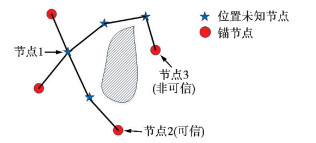图 3 可信锚节点判断 Fig.3 Trusted anchor node judgment

3.1 稀疏锚节点网络中可信锚节点选择阈值的确定

 $hl = r\left( {1 + {{\rm{e}}^{ - \rho }} - \int_{ - 1}^1 {{{\rm{e}}^{\frac{\rho }{\pi }({\rm{arccost}} - t\sqrt {1 - {t^2}} )}}} {\rm{d}}t} \right)$ (5)

 $\left\{ {\begin{array}{*{20}{l}} {h = 1,h{l_{{\rm{min}}}} = 0}\\ {h = 2,h{l_{{\rm{min}}}} = \frac{r}{2}}\\ {h = 3,h{l_{{\rm{min}}}} = \frac{{r + hl}}{3}}\\ {h = k,h{l_{{\rm{min}}}} = \frac{{r + (k - 2)hl}}{k},k \ge 3} \end{array}} \right.$ (6)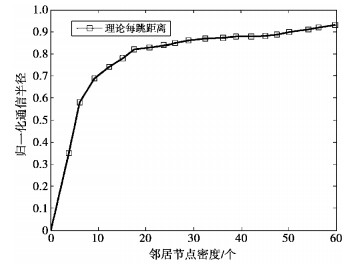图 4 不同邻居节点密度下理论平均每跳距离 Fig.4 Theoretical average hop distance for different neighbor node densities
3.2 稀疏锚节点网络中可信锚节点的选择

1：for i=1:kjA

2：Reliable_anchor_set(i)=Neighbor_anchor_set(i);

3：确定节点iρi，利用(5)式计算hl(i);

4：threshold=lookup(hl(i), hij);

5：if Neighbor_anchor_set(i)=1 (邻居锚节点A1)

6：节点i距离锚节点j可能的最短距离坐标X1(x1i, y1i);

7：计算X1与锚节点j之间的欧氏距离dshort ;

8：end if

9：if Neighbor_anchor_set(i)=2 (邻居锚节点A1A2)

10：确定圆C1与圆C2交点坐标X1(x1i, y1i)和X2(x2i, y2i);

11：计算交点与锚节点j之间的欧氏距离d1d2;

12：dshort=min{d1, d2};

13：end if

14：hlshort=dshort/hij;

15：if hlshort> threshold

16：reliable[j]=true;

17：Reliable_anchor_set(i)=j;

18：end if

19：end for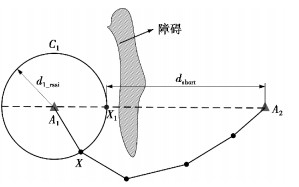图 5 n(i)=1时，未知节点可能的位置情况 Fig.5 Possible position of unknown node when n(i)=1

 $h{l_{{\rm{ short }}}} = {d_{{\rm{ short }}}}/h$ (7)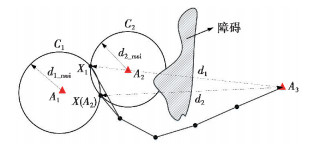图 6 n(i)=2时，未知节点可能的位置情况 Fig.6 When n(i)=2, the possible location of the unknown node
3.3 基于节点定位的最佳路径搜索

1：未知节点通过Flooding路由方式记录与之通信锚节点的坐标及最小跳数;

2：for i=1: Mini_hop-1

4：  if i=1(目标节点开始)

5：    搜索节点的邻居节点，使邻居节点到出口锚节点跳数满足Mini_hop-i;

6：  else if i>1 (目标节点邻居节点开始)

7：    搜索节点的邻居节点，使其到出口锚节点跳数满足Mini_hop-i;

8：   end if

10：end for

14：end for

4 移动节点定位导航

 $\left\{ {\begin{array}{*{20}{l}} {{x_t} = {x_{t - 1}} + {s_{t - 1,t}} \cdot {\rm{cos}}{\varphi _{t - 1}}}\\ {{y_t} = {y_{t - 1}} + {s_{t - 1,t}} \cdot {\rm{sin}}{\varphi _{t - 1}}}\\ {{\varphi _{t - 1}} = {\varphi _t} - {\theta _{t - 1,t}}} \end{array}} \right.$ (8)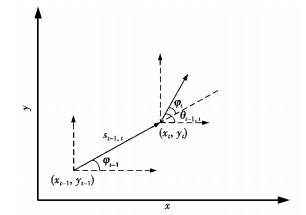图 7 历史信息节点估计算法 Fig.7 Historical information node estimation algorithm

5 仿真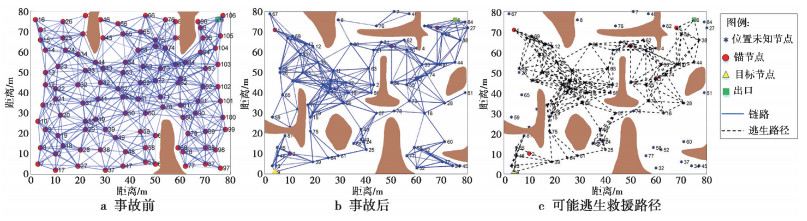图 8 可能逃生救援路径生成的网络拓扑变化仿真 Fig.8 Simulation of network topology changes generated by possible escape rescue paths
5.1 可信锚节点可信判断与定位误差分析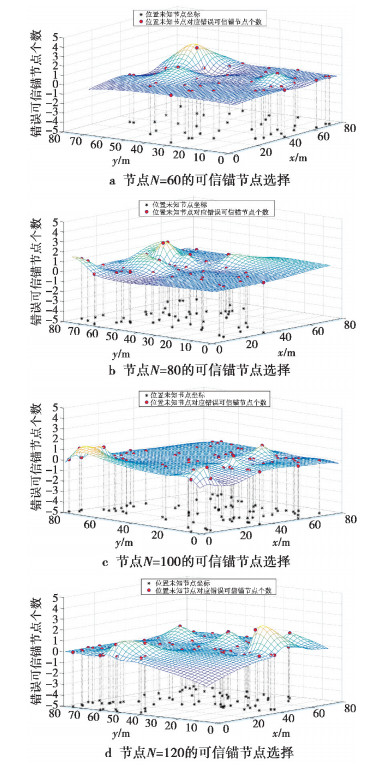图 9 第一次迭代可信锚节点集准确性判断 Fig.9 Accuracy judgment of the set of trusted anchor nodes in the first iteration

 $e=\frac{\sum\limits_{i=1}^{m}{\sqrt{{{({{x}_{i}}-{{x}^{'}}_{i})}^{2}}+{{({{y}_{i}}-{{y}^{'}}_{i})}^{2}}}}}{mr}$ (9)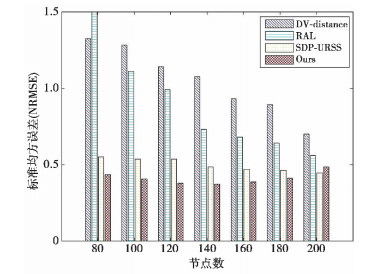图 10 不同节点数NRMSE计算结果 Fig.10 NRMSE calculation results for different node numbers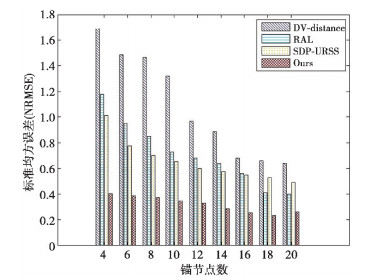图 11 不同锚节点NRMSE计算结果 Fig.11 NRMSE calculation results for different anchor nodes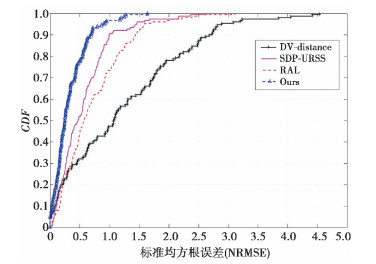图 12 NRMSE的CDF分布图 Fig.12 CDF distribution of NRMSE
5.2 最佳路径确定及受困人员移动导航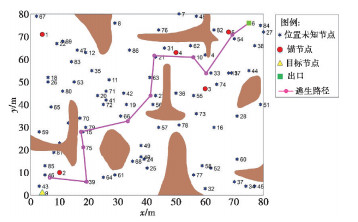图 13 最终路线图，NRMSE=0.31r Fig.13 Final route, NRMSE=0.31r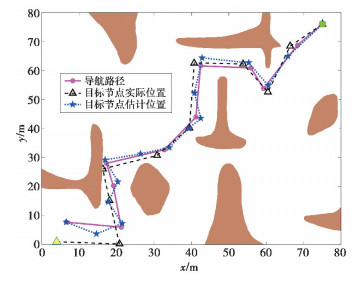图 14 导航结果 Fig.14 Navigation result
6 结束语

  白云, 侯媛彬. 煤矿救援蛇形机器人的研制与控制[J]. 西安科技大学学报, 2018(05): 800-808. BAI Y, HOU Y B. Research on environment modeling method of the coal mine rescue snake-like robot[J]. Journal of Xi'an University of Science and Technology, 2018(05): 800-808.  张先华, 李政, 谭小慧, 等. 基于灰色马尔科夫的地铁火灾逃生最优路径规划及3D模拟[J]. 重庆理工大学学报(自然科学), 2019, 33(8): 118-123. ZHANG Xianhua, LI Zheng, TAN Xiaohui, et al. Based on Grey Markov Prediction Algorithm Metro Fire Escape Route Planning and 3D Simulation[J]. Journal of Chongqing University of Technology(Natural Science), 2019, 33(8): 118-123.  董梦梦, 王翥. 面向机场环境监测的WSN组网技术[J]. 江苏大学学报(自然科学版), 2016, 37(5): 578-584. DONG M M, WANG Z. WSN networking technology for airport environment monitoring[J]. Journal of Jiangsu University (Natural Science Edition), 2016, 37(5): 578-584. DOI:10.3969/j.issn.1671-7775.2016.05.014  HE T, HUANG C, BLUM B M, et al. Range-free localization schemes for large scale sensor networks[C]//Proceedings of the 9th annual international conference on Mobile computing and networking.San Diego, CA, USA: ACM, 2003: 81-95.  冯立, 姚远程, 胡荣春. 基于分层定位的无线传感器网络定位研究[J]. 重庆邮电大学学报(自然科学版), 2008, 20(4): 427-430. FENG L, YAO Y C, HU R C. Research on wireless sensor localization based on hierarchical localization[J]. Journal of Chongqing University of Posts and Telecommunications (Natural Science Edition), 2008, 20(4): 427-430.  ESPINOZA A, REYES E, RUIZ E, et al. Performance Comparison Between Simulated and Real Case Scenario of RSSI Based Localization Algorithms on a WSAN[J]. IEEE Latin America Transactions, 2016, 14(1): 115-121.  AHMADI H, VIANI F, BOUALLEGUE R. An accurate prediction method for moving target localization and tracking in wireless sensor networks[J]. Ad Hoc Networks, 2018(70): 14-22.  SHAHZAD F, SHELTAMI T R, SHAKSHUKIE M. DV-maxHop: A fast and accurate range-free localization algorithm for anisotropic wireless networks[J]. IEEE Transactions on Mobile Computing, 2016, 16(9): 2494-2505.  XIAO B, CHEN L, XIAO Q, et al. Reliable anchor-based sensor localization in irregular areas[J]. IEEE Trans Mobile Comput, 2010, 9(1): 60-72. DOI:10.1109/TMC.2009.100  刘洁琳.基于无线传感网络的室内移动节点定位技术研究[D].沈阳: 沈阳理工大学, 2018. LIU J L. Research on Indoor Mobile Node Location Technology Based on Wireless Sensor Network[D]. Shenyang: Shenyang Institute of Technology, 2018. http://cdmd.cnki.com.cn/Article/CDMD-10144-1018141447.htm  WILLIS S, KIKKERT C J. Radio propagation model for long-range wireless sensor networks[C]//2007 6th International Conference on Information, Communications & Signal Processing. Singapore: IEEE, 2007: 1-5.  XIAO Q, XIAO B, CAO J, et al. Multihop range-free localization in anisotropic wireless sensor networks: A pattern-driven scheme[J]. IEEE Trans Mobile Comput, 2010, 9(11): 1592-1607. DOI:10.1109/TMC.2010.129  蓝威涛, 张卫强, 罗健宇. 一种自适应智能三边定位算法的设计与实现[J]. 传感技术学报, 2017, 30(07): 1089-1094. LAN W T, ZHANG W Q, LUO J Y. Design and Implementation of an Adaptive Intelligent Triangulation Algorithm[J]. Journal of Sensing Technology, 2017, 30(07): 1089-1094. DOI:10.3969/j.issn.1004-1699.2017.07.020  张宏刚, 黄华. 基于RSSI路径损耗因子动态修正的三边质心定位算法[J]. 传感技术学报, 2016, 29(11): 1731-1736. ZHANG H G, HUANG H. Trilateral centroid localization algorithm based on dynamic correction of RSSI path loss factor[J]. Journal of Sensing Technology, 2016, 29(11): 1731-1736. DOI:10.3969/j.issn.1004-1699.2016.11.017  罗雯雯.无线传感器网络自主移动节点定位技术研究[D].浙江: 浙江理工大学, 2013. LUO W W. Research on Autonomous Mobile Node Location Technology in Wireless Sensor Networks[D]. Zhejiang: Zhejiang University of Technology, 2013. http://cdmd.cnki.com.cn/Article/CDMD-10338-1013290560.htm  王福豹, 史龙, 任丰原. 无线传感器网络中的自身定位系统和算法[J]. 软件学报, 2005, 16(5): 857-868. WANG F B, SHI L, REN F Y. Self-positioning system and algorithm in wireless sensor networks[J]. Journal of Software, 2005, 16(5): 857-868.  OUYANG R W, WONG A K S, LEA C T. Received signal strength based wireless localization via semidefinite programming: Noncooperative and cooperative schemes[J]. IEEE Trans Veh Technol, 2010, 59(3): 1307-1318.